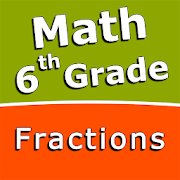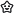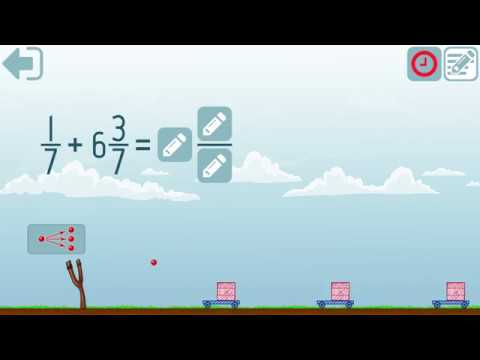# Fractions and mixed numbers - 6th grade mathEveryoneFamily FriendlyIntuitive interface powered by handwriting input and a choice of three fun and engaging mini games in addition to a regular math trainer mode make our app stand out from the crowd of generic math learning apps.

With Fractions and mixed numbers - 6th grade math you can practice and improve the following math skills:
- Write fractions in lowest terms
- Add fractions and mixed numbers with like denominators
- Add fractions and mixed numbers with unlike denominators
- Subtract fractions and mixed numbers with like denominators
- Subtract fractions and mixed numbers with unlike denominators
- Multiply fractions and mixed numbers
- Multiply mixed numbers and whole numbers
- Divide fractions and mixed numbers
- Divide mixed numbers by whole numbers
- Convert fractions and mixed numbers to decimals

Choose between four different modes:
- Math Trainer
- Math Shot mini-game
- Math Gravity mini-game
- Math Trick Shot mini-game
Collapse

## Reviews

Review Policy

Eligible for Family LibraryEligible if bought after 7/2/2016. Learn More
Updated
December 9, 2018
Size
11M
Installs
100+
Current Version
1.0.0
Requires Android
4.0 and up
Content Rating
Everyone
Permissions
Offered By
Sergey Malugin
Developer
Taubenbrunnen 6 37120 Bovenden Germany